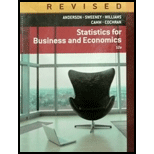Chapter 1, Problem 24SEStatistics for Business & Economic...

12th Edition
David R. Anderson + 4 others
ISBN: 9781285846323

Solutions

Chapter
SectionStatistics for Business & Economic...

12th Edition
David R. Anderson + 4 others
ISBN: 9781285846323
Textbook Problem

A sample of midterm grades for five students showed the following results: 72, 65, 82, 90, 76. Which of the following statements are correct, and which should be challenged as being too generalized? a. The average midterm grade for the sample of five students is 77. b. The average midterm grade for all students who took the exam is 77. c. An estimate of the average midterm grade for all students who took the exam is 77. d. More than half of the students who take this exam will score between 70 and 85. e. If five other students are included in the sample, their grades will be between 65 and 90.

a.

To determine

Find whether the statement “The average midterm grade for the sample of five students is 77” is correct or too generalized.

Explanation

Calculation:

The given information is that the sample consists of five students whose midterm grades are 72, 65, 82, 90, and 76.

In the statement, the average of the midterm grades for the sample is 77.

The average value is obtained as given below:

Average=72+

b.

To determine

Find whether the statement “The average midterm grade for all students who took the exam is 77” is correct or too generalized.

c.

To determine

Find whether the statement “An estimate of the average midterm grade for all students who took the exam is 77” is correct or too generalized.

d.

To determine

Find whether the statement “More than half of the students who take this exam will score between 70 and 85” is correct or too generalized.

e.

To determine

Find whether the statement “If five other students are included in the sample, their grades will be between 65 and 90” is correct or too generalized.

Still sussing out bartleby?

Check out a sample textbook solution.

See a sample solution

The Solution to Your Study Problems

Bartleby provides explanations to thousands of textbook problems written by our experts, many with advanced degrees!

Get Started

In what ways is economics a science?

Essentials of Economics (MindTap Course List)

BOND VALUATION You are considering a 10-year, 1,000 par value bond. Its coupon rate is 9%, and interest is paid...

Fundamentals of Financial Management, Concise Edition (with Thomson ONE - Business School Edition, 1 term (6 months) Printed Access Card) (MindTap Course List)

How does SCM software support SCM?

Pkg Acc Infor Systems MS VISIO CD

PAYBACK PERIOD Refer to Problem 11-1. What is the projects payback?

Fundamentals of Financial Management (MindTap Course List)